﻿ 考虑刚柔耦合效应的风力机叶片动力学分析 Dynamic Analysis of Wind Turbine Blade with the Rigid-Flexible Coupling Effect

Hans Journal of Civil Engineering
Vol.07 No.02(2018), Article ID:24135,9 pages
10.12677/HJCE.2018.72025

Dynamic Analysis of Wind Turbine Blade with the Rigid-Flexible Coupling Effect

Kaiqiang Gao, Zhiqiang Zhang

School of Civil Engineering, Southeast University, Nanjing Jiangsu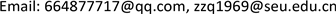Received: Mar. 2nd, 2018; accepted: Mar. 16th, 2018; published: Mar. 23rd, 2018ABSTRACT

To analyze the vibrational frequencies and dynamic response of wind turbine blade with wind loads, the zeroth-order approximation model was adopted and the centrifugal stiffening effect was included to derive the dynamic equation of the rotating blade. The formula of stiffness matrix and mass matrix was given in the paper. Finally, the vibrational frequency and wind-induced response of a blade were calculated. The results show that the rigid-flexible coupling effect has few effects on the out-plane stiffness of blade. The in-plane stiffness of blade, however, was affected by this effect. The centrifugal stiffening effect both strengthened the in-plane and out-plane stiffness of blade. The results of the paper have some reference value to the dynamic design of wind turbine blades.

Keywords:Dynamics of Blade, Rigid-Flexible Coupling Effect, Zeroth-Order Approximation Model, Centrifugal Stiffening Effect1. 引言

2. 柔性梁的动力有限元方程

2.1. 刚柔耦合建模理论

${u}_{f}={\left\{{u}^{i},{v}^{i},{w}^{i},{\theta }_{1}^{i},{\theta }_{2}^{i},{\theta }_{3}^{i},{u}^{j},{v}^{j},{w}^{j},{\theta }_{1}^{j},{\theta }_{2}^{j},{\theta }_{3}^{j}\right\}}^{\text{T}}$ (1)

$\begin{array}{l}{u}_{k}={N}_{1}\left({\xi }_{k}\right){u}_{f},{v}_{k}={N}_{2}\left({\xi }_{k}\right){u}_{f},{w}_{k}={N}_{3}\left({\xi }_{k}\right){u}_{f},\\ {\theta }_{1k}={N}_{4}\left({\xi }_{k}\right){u}_{f},{\theta }_{2k}=-\frac{\partial {w}_{k}}{\partial \xi }=-\frac{\partial {N}_{3}\left({\xi }_{k}\right)}{\partial \xi }{u}_{f},{\theta }_{3k}=\frac{\partial {w}_{k}}{\partial \xi }=\frac{\partial {N}_{2}\left({\xi }_{k}\right)}{\partial \xi }{u}_{f}\end{array}$ (2)

$\begin{array}{l}{N}_{1}=\left[{a}_{1},0,0,0,0,0,{a}_{2},0,0,0,0,0\right],\\ {N}_{2}=\left[0,{b}_{1},0,0,0,{b}_{2},0,{b}_{3},0,0,0,{b}_{4}\right],\\ {N}_{3}=\left[0,0,{b}_{5},0,{b}_{6},0,0,0,{b}_{7},0,{b}_{8},0\right],\\ {N}_{4}=\left[0,0,0,{a}_{1},0,0,0,0,{a}_{2},0,0,0\right],\end{array}$ (3)

$\begin{array}{l}{a}_{1}=1-\xi ,\text{\hspace{0.17em}}{a}_{2}=\xi ,\text{\hspace{0.17em}}{b}_{1}=1-3{\xi }^{2}+2{\xi }^{3},\\ {b}_{2}=l\left(\xi -2{\xi }^{2}+{\xi }^{3}\right),\text{\hspace{0.17em}}{b}_{3}=3{\xi }^{2}-2{\xi }^{3},\\ {b}_{4}=l\left(-{\xi }^{2}+{\xi }^{3}\right),\text{\hspace{0.17em}}{b}_{5}=1-3{\xi }^{2}+2{\xi }^{3},\\ {b}_{6}=l\left(-\xi +2{\xi }^{2}-{\xi }^{3}\right),\text{\hspace{0.17em}}{b}_{7}=3{\xi }^{2}-2{\xi }^{3},\\ {b}_{8}=l\left(2{\xi }^{2}-{\xi }^{3}\right)\end{array}$ (4)

$r={r}_{0}+A\left({\rho }_{0}+{p}_{k}\right)$ (5)

$\begin{array}{l}{r}_{0}={\left[R\mathrm{cos}\omega t,R\mathrm{sin}\omega t,0\right]}^{\text{T}},\text{\hspace{0.17em}}{\rho }_{0}={\left[x,y,z\right]}^{\text{T}}\\ A=\left[\begin{array}{ccc}\mathrm{cos}\omega t& -\mathrm{sin}\omega t& 0\\ \mathrm{cos}\omega t& \mathrm{cos}\omega t& 0\\ 0& 0& 1\end{array}\right]\\ {p}_{k}=\left[\begin{array}{c}{N}_{1}-y\frac{\partial {N}_{2}}{\partial \xi }-z\frac{\partial {N}_{3}}{\partial \xi }\\ \begin{array}{l}{N}_{2}-z{N}_{4}\\ {N}_{3}+y{N}_{4}\end{array}\end{array}\right]{u}_{k}\end{array}$ (6)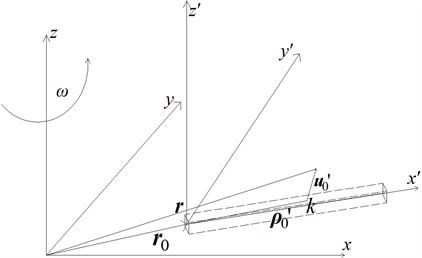Figure 1. The beam rotates around a fixed axis

$\begin{array}{l}{v}_{k}=\stackrel{˙}{r}={\stackrel{˙}{r}}_{0}+A\stackrel{˜}{\alpha }\left({\rho }_{0}+{p}_{k}\right)+A{\stackrel{˙}{p}}_{k}\\ {a}_{k}={\stackrel{¨}{r}}_{0}+\left(\stackrel{˜}{\stackrel{˙}{\alpha }}A+\stackrel{˜}{\alpha }\stackrel{˜}{\alpha }A\right)\left({\rho }_{0}+{p}_{k}\right)+2\stackrel{˜}{\alpha }A{\stackrel{˙}{p}}_{k}+A{\stackrel{¨}{p}}_{k}\end{array}$ (7)

$\stackrel{˜}{\alpha }=\left[\begin{array}{ccc}0& -\omega & 0\\ \omega & 0& 0\\ 0& 0& 0\end{array}\right]$ (8)

${f}^{*}+f=0$ (9)

${f}^{*}={\left\{\frac{\partial {v}_{k}}{\partial \stackrel{˙}{u}}\right\}}^{\text{T}}{F}^{*},\text{\hspace{0.17em}}{F}^{*}=-\rho \cdot {a}_{k}\text{d}v$ (10)

f为作用在微元体上的其他广义惯性力，应该包括该点的外力，弹性恢复力，阻尼力以及结构的内力。

$M\stackrel{¨}{u}+C\stackrel{˙}{u}+Ku=Q$ (11)

2.2. 离心刚化效应

$\text{d}s-\text{d}x=\sqrt{1+{\left(\frac{\partial V}{\partial x}\right)}^{2}}\text{d}x-\text{d}x\approx \frac{1}{2}{\left(\frac{\partial V}{\partial x}\right)}^{2}\text{d}x$ (12)

${U}_{exy}=\frac{1}{2}\underset{0}{\overset{L}{\int }}F\left(x\right){\left(\frac{\partial V}{\partial x}\right)}^{2}\text{d}x$ (13)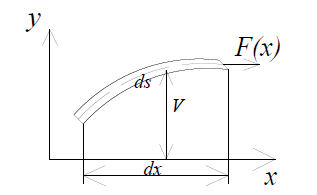Figure 2. The bending of flexible beam in the xy plane

$F\left(x\right)=\underset{x}{\overset{L}{\int }}\rho {\omega }^{2}\left(x+R\right)\text{d}V=\underset{x}{\overset{L}{\int }}\rho A\left(x\right){\omega }^{2}\left(x+R\right)\text{d}x$ (14)

${U}_{exz}=\frac{1}{2}\underset{0}{\overset{L}{\int }}F\left(x\right){\left(\frac{\partial W}{\partial x}\right)}^{2}\text{d}x$ (15)

${U}_{e}=\frac{1}{2}\underset{0}{\overset{L}{\int }}F\left(x\right)\left[{\left(\frac{\partial V}{\partial x}\right)}^{2}+{\left(\frac{\partial W}{\partial x}\right)}^{2}\right]\text{d}x$ (16)

$\begin{array}{c}{K}_{e}=\underset{0}{\overset{{l}_{1}}{\int }}F\left(\xi \right)\left(\frac{\partial {N}_{2}^{\text{T}}}{\partial \xi }\frac{\partial {N}_{2}}{\partial \xi }+\frac{\partial {N}_{3}^{\text{T}}}{\partial \xi }\frac{\partial {N}_{3}}{\partial \xi }\right)\text{d}\xi \\ \text{\hspace{0.17em}}\text{\hspace{0.17em}}+\sum _{i=2}^{n}\underset{0}{\overset{{l}_{i}}{\int }}F\left(\xi +\sum _{j=1}^{i-1}{l}_{j}\right)\left(\frac{\partial {N}_{2}^{\text{T}}}{\partial \xi }\frac{\partial {N}_{2}}{\partial \xi }+\frac{\partial {N}_{3}^{\text{T}}}{\partial \xi }\frac{\partial {N}_{3}}{\partial \xi }\right)\text{d}\xi \end{array}$ (17)

3. 叶片的旋转固有频率分析

$M\stackrel{¨}{u}={\beta }^{2}Ku$ (18)

$\begin{array}{l}M=\sum _{i=1}^{n}\rho \underset{vi}{\int }{N}_{1}^{\text{T}}{N}_{1}+{N}_{2}^{\text{T}}{N}_{2}+{N}_{3}^{\text{T}}{N}_{3}+\left({y}^{2}+{z}^{2}\right){N}_{4}^{\text{T}}{N}_{4}\text{d}V\\ \text{\hspace{0.17em}}\text{\hspace{0.17em}}\text{\hspace{0.17em}}\text{\hspace{0.17em}}\text{\hspace{0.17em}}\text{\hspace{0.17em}}\text{\hspace{0.17em}}\text{ }+\sum _{i=1}^{n}\rho \underset{vi}{\int }{y}^{2}\frac{\partial {N}_{2}^{\text{T}}}{\partial \overline{x}}\frac{\partial {N}_{2}}{\partial \overline{x}}+{z}^{2}\frac{\partial {N}_{3}^{\text{T}}}{\partial \overline{x}}\frac{\partial {N}_{3}}{\partial \overline{x}}\text{d}V\\ K=\sum _{i=1}^{n}{K}_{fi}+{K}_{d},\\ {K}_{d}=-\sum _{i=1}^{n}{\omega }^{2}\underset{vi}{\int }{N}_{1}^{\text{T}}{N}_{1}+{N}_{2}^{\text{T}}{N}_{2}+{z}^{2}{N}_{4}^{\text{T}}{N}_{4}\text{d}V\\ \text{\hspace{0.17em}}\text{\hspace{0.17em}}\text{\hspace{0.17em}}\text{\hspace{0.17em}}\text{\hspace{0.17em}}\text{\hspace{0.17em}}\text{\hspace{0.17em}}\text{\hspace{0.17em}}-\sum _{i=1}^{n}{\omega }^{2}\underset{vi}{\int }{y}^{2}\frac{\partial {N}_{2}^{\text{T}}}{\partial \overline{x}}\frac{\partial {N}_{2}}{\partial \overline{x}}+{z}^{2}\frac{\partial {N}_{3}^{\text{T}}}{\partial \overline{x}}\frac{\partial {N}_{3}}{\partial \overline{x}}\text{d}V\end{array}$ (19)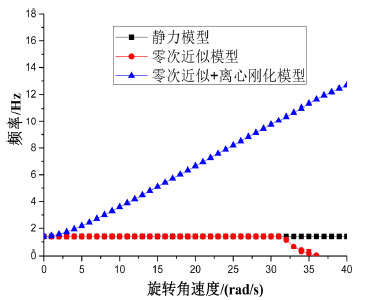Figure 3. The first natural frequency varied with the change of rotation speed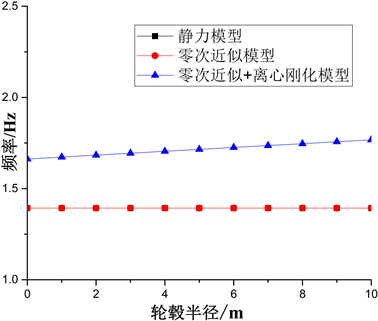Figure 4. The first natural frequency varied with the change of radius of hub

1) 在标准工况下，零次近似模型的1、2、4、5阶频率与静力模型几乎完全相同。而它的3阶频率比静力模型要小，此时叶片的1、2、4、5阶频率是z向(旋转面外)弯曲，3阶频率是y向(旋转面内)弯曲。则说明Kd刚度阵对叶片旋转面内的刚度具有弱化作用，而对旋转面外的刚度影响微乎其微。

2) 在标准工况下，零次近似 + 离心刚化模型的前5阶频率都要比静力和零次近似模型大，说明Ke刚度阵对叶片旋转面内和面外都产生刚化作用，而且在面内盖过了Kd矩阵弱化的影响。

3) 观察叶片频率随轮毂半径的变化可知，零次近似 + 离心刚化模型随着轮毂半径的增大而增大，这是因为Ke矩阵的表达式与轮毂半径R有关，且轮毂半径越大，它们对叶片所起的刚化作用越强。而Kd矩阵与轮毂半径无关，所以零次近似模型和静力模型相同。

4. 叶片随风动力响应分析

4.1. 风荷载计算

${f}_{w}=\frac{1}{2}{C}_{d}{\rho }_{\text{air}}B{\left({u}_{m}+{u}_{f}\right)}^{2}$ (20)

${u}_{m}={V}_{h}{\left(\frac{r\cdot \mathrm{sin}\left({\omega }_{0}t\right)+h}{h}\right)}^{\alpha }$ (21)

4.2. 叶片随风振动分析

1) 零次近似模型和静力模型计算的结果基本一致，这是因为Kd刚度阵只对旋转面内的刚度有影响。

2) 零次近似 + 离心刚化模型的z向位移相比静力模型要小，这说明Ke矩阵对叶片旋转面外的刚度具有增强效果，能够降低叶片在随机风载作用下的位移。Figure 5. The time history curves of wind-induced response of blade tip under different models

5. 结论

1) 叶片频率的研究表明，Kd对叶片的旋转面内刚度产生软化作用，降低了叶片的振动频率，且易使得叶片在旋转面内的弯曲变形增大。当转速达到一定程度时，结构便遭到了破坏。而Ke对叶片旋转面内和面外的刚度均具有增强效果，提高了结构的振动频率。

2) 叶片随风振动的分析表明，零次近似模型的计算结果和静力模型基本相同，而零次近似 + 离心刚化模型的风振响应较前两者要小，说明了Kd刚度矩阵对叶片的面外刚度不产生影响作用，而离心刚化效应使叶片的面内外刚度都得到了增强，所以能够降低叶片在随机风载作用下的位移。因此，今后的叶片风振响应分析当中(即垂直于平面的运动)，可以不考虑Kd的影响，而叶片的离心刚化效应则需要加以考虑。

Dynamic Analysis of Wind Turbine Blade with the Rigid-Flexible Coupling Effect[J]. 土木工程, 2018, 07(02): 205-213. https://doi.org/10.12677/HJCE.2018.72025

1. 1. Putter, S. and Manor, H. (1978) Natural Frequencies of Radial Rotating Beams. Journal of Sound and Vibration, 56, 175-185. https://doi.org/10.1016/S0022-460X(78)80013-3

2. 2. Yoo, H.H., Park, J.H. and Park, J.H. (2001) Vibration Analysis of Rotat-ing Pre-Twisted Blades. Computers and Structures, 79, 1811-1819. https://doi.org/10.1016/S0045-7949(01)00110-9

3. 3. Choi, D.H., Park, J.H. and Yoo, H.H. (2004) Modal Analysis of Constrained Multibody Systems Undergoing Rotational Motion. Journal of Sound and Vibration, 280, 63-76. https://doi.org/10.1016/j.jsv.2003.12.011

4. 4. 李德源, 叶枝全, 包能胜, 等. 风力机旋转风轮振动模态分析[J]. 太阳能学报, 2004, 25(1): 72-77.

5. 5. 孙保苍, 李鹏飞. 考虑应力刚化影响的风力机叶片振动模态分析[J]. 可再生能源, 2012, 30(5): 38-41.

6. 6. 信伟平. 风力机旋转叶片动力特性及响应分析[D]: [硕士学位论文]. 汕头: 汕头大学, 2005.

7. 7. 柯世堂, 曹九发, 王珑, 等. 风力机塔架-叶片耦合模型风致响应时域分析[J]. 湖南大学学报(自然科学版), 2014, 41(4): 87-93.

8. 8. 柯世堂, 王同光, 胡丰, 等. 基于塔架-叶片耦合模型风力机全机风振疲劳分析[J]. 工程力学, 2015, 32(8): 36-41.

9. 9. 洪嘉振. 计算多体系统动力学[M]. 北京: 高等教育出版社, 2003: 60-62.

10. 10. 蹇开林, 殷学纲. 旋转梁的固有频率计算[J]. 重庆大学学报: 自然科学版, 2001, 24(6): 36-39.

11. 11. 吴艳红. 旋转叶片刚柔耦合系统动力学研究[D]: [博士学位论文]. 哈尔滨: 哈尔滨工程大学, 2011.

12. 12. Naguleswaran, S. (1994) Lateral Vibration of a Centrifugally Tensioned Uniform Eu-ler-Bernoulli Beam. Journal of Sound and Vibration, 176, 613-624. https://doi.org/10.1006/jsvi.1994.1402

13. 13. 王勖成. 有限单元法[M]. 北京: 清华大学出版社, 2003: 28-36.

14. 14. Murtagh, P.J., Basu, B. and Broderick, B.M. (2005) Along-Wind Response of a Wind Turbine Tower with Blade Coupling Subject to Rotationally Sampled Wind Loading. Engineering Structure, 27, 1209-1219. https://doi.org/10.1016/j.engstruct.2005.03.004

15. 15. 陈小波, 陈健云, 李静. 海上风力发电塔脉动风速程数值模拟[J]. 中国电机工程学报 2008, 28(32): 111-116.